Question 9

For the three events A, B, C,

P(exactly one of A or B occurs) = P(exactly one of B or C occurs) = P(exactly one of C or A occurs) = p and P(all the three events occur simultaneously =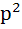where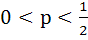. Then the probability of at least one of A, B, C occurring is

a)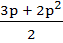b)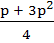c)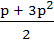d)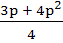IIT 1996
 Your Answer ABCD CLEAR « Previous Answer: a Next »